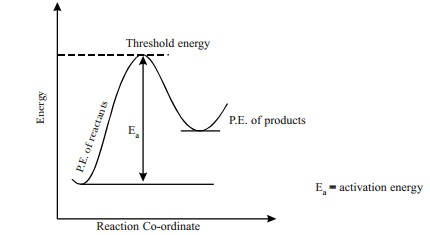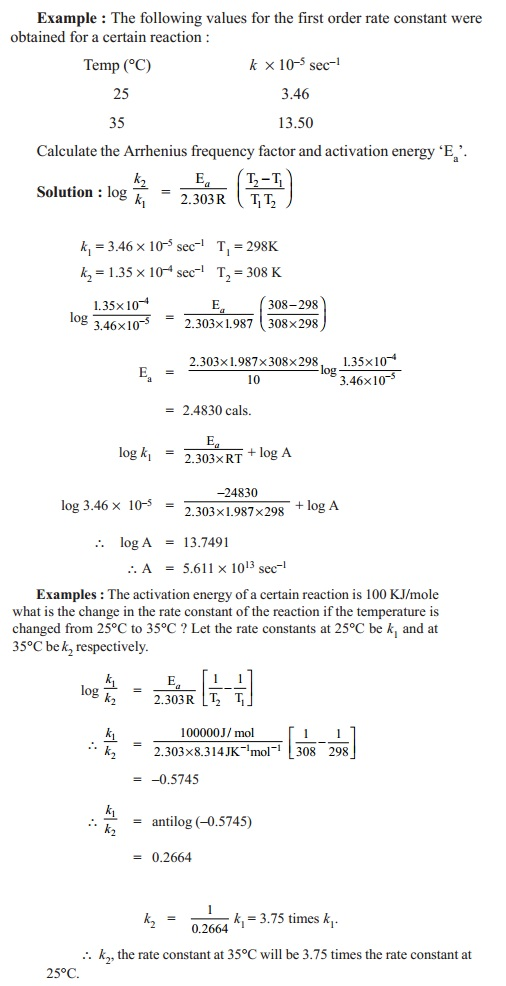Home | | Chemistry | Temperature Dependance Of Rate Constant

# Temperature Dependance Of Rate ConstantIt is a common observation that rates of reactions increase with increase in temperature of the reaction mixture. Keeping the concentration of the reactants constant, the rate is found to be two times its initial value, when measured at a temperature 10 K greater than the initial temperature.

TEMPERATURE DEPENDANCE OF RATE CONSTANT

It is a common observation that rates of reactions increase with increase in temperature of the reaction mixture. Keeping the concentration of the reactants constant, the rate is found to be two times its initial value, when measured at a temperature 10 K greater than the initial temperature. However, the exact value of the rate constant determined at various temperature is predicted by using Arrhenius equation. This expression is obeyed by most of the reactions. Arrhenius equation is given as

k = A e-Ea/RT

where k = rate constant, Ea = activation energy, A = frequency factor, R = gas constant, T = temperature in Kelvin. If k1 and k2 are the rate constants measured at two different T1 and T temperatures respectively, then Ea can be determined as follows :

Arrhenius equation for two different temperatures T1 and T2 are :

log k1 = log A - (Ea / 2. 303R T1)

log k2 = log A - (Ea / 2. 303R T2)

where k1 and k2 are the rate constants at temperature T1 and T2 respectively.

log k2 - log k1= - (Ea / 2. 303R T2) + (Ea / 2. 303R T1)

log(k2 /k2) =  Ea / 2. 303R ( T2-T1 /  T1T2)

If  R = 1.987 cals.mol-1 , then unit of E is 10-3  k.cal.

Also, a plot of log k against 1/T values gives a straight line with slope value equals to -E a/2.303 R and intercept value equals to log A. When Ea is a positive value, and if T2 > T1 then k2 > k1. That is, rate constant value at higher temperature is greater than rate constant value at lower temperature. Under such conditions, plot of log k against 1/T gives a negative slope straight line. From the slope of the straight line, Ea can be calculated.Reactant molecules come into contact through collisions for transformation into product molecules. Since, not all collisions are successful in producing the product molecules, all colliding molecules must possess certain minimum energy called as the threshold energy which is needed to make the collisions effective and successful. The additional energy required by the molecules to attain the threshold energy in addition to the energy of colliding molecules is called as activation energy 'Ea'. Thus, activation energy = threshold energy - Energy of colliding molecules. Generally, this Ea value is higher than the potential energies of reactants and products. Thus, Ea is considered as an energy barrier that must be crossed by the reactant molecules before getting converted to actual product molecules.Potential energy diagram of a reaction. The molecular state present at the Ea position in the potential energy diagram, is considered as the intermediate product (or) the transition state. Thus Ea, is also the energy required to form the activated state or the intermediate, which is necessary to form the products. Ea is a characteristic value of a reaction. The rate, rate constant, and their temperature dependance are determined by the value of Ea. Higher the value of Ea, slower is the rate of the reaction.Study Material, Lecturing Notes, Assignment, Reference, Wiki description explanation, brief detail
11th 12th std standard Class Organic Inorganic Physical Chemistry Higher secondary school College Notes : Temperature Dependance Of Rate Constant |